Next: Newton's third law of Up: Newtonian mechanics Previous: Newton's first law of

# Newton's second law of motion

Newton's second law of motion essentially states that if a point object is subject to an external force,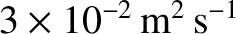, then its equation of motion is given by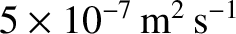(2.13)

where the momentum,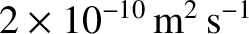, is the product of the object's inertial mass,, and its velocity,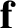. Ifis not a function of time then Equation (2.13) reduces to the familiar equation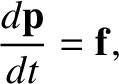(2.14)

Note that this equation is only valid in a inertial frame. Clearly, the inertial mass of an object measures its reluctance to deviate from its preferred state of uniform motion in a straight line (in an inertial frame). Of course, the preceding equation of motion can only be solved if we have an independent expression for the force,(i.e., a law of force). Let us suppose that this is the case.

An important corollary of Newton's second law is that force is a vector quantity. This must be the case, because the law equates force to the product of a scalar (mass) and a vector (acceleration).2.2 Note that acceleration is obviously a vector because it is directly related to displacement, which is the prototype of all vectors. One consequence of force being a vector is that two forces,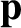and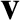, both acting at a given point, have the same effect as a single force,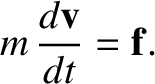, acting at the same point, where the summation is performed according to the laws of vector addition. Likewise, a single force,, acting at a given point, has the same effect as two forces,and, acting at the same point, provided that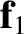. This method of combining and splitting forces is known as the resolution of forces; it lies at the heart of many calculations in Newtonian mechanics.

Taking the scalar product of Equation (2.14) with the velocity,, we obtain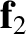(2.15)

This can be written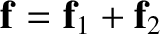(2.16)

where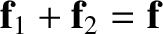(2.17)

The right-hand side of Equation (2.16) represents the rate at which the force does work on the object; that is, the rate at which the force transfers energy to the object. The quantityrepresents the energy that the object possesses by virtue of its motion. This type of energy is generally known as kinetic energy. Thus, Equation (2.16) states that any work done on point object by an external force goes to increase the object's kinetic energy.

Suppose that, under the action of the force,, our object moves from point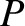at time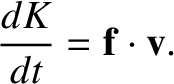to point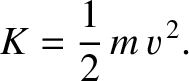at time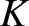. The net change in the object's kinetic energy is obtained by integrating Equation (2.16):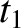(2.18)

because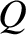. Here,is an element of the object's path between pointsand, and the integral in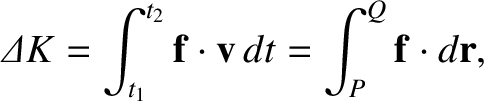represents the net work done by the force as the object moves along the path fromto.

As is well known, there are basically two kinds of forces in nature: first, those for which line integrals of the type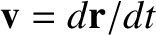depend on the end points, but not on the path taken between these points; second, those for which line integrals of the typedepend both on the end points, and the path taken between these points. The first kind of force is termed conservative, whereas the second kind is termed non-conservative. It can be demonstrated that if the line integralis path-independent, for all choices ofand, then the forcecan be written as the gradient of a scalar field. (See Section A.5.) In other words, all conservative forces satisfy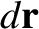(2.19)

for some scalar field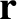. [Incidentally, mathematicians, as opposed to physicists and astronomers, usually write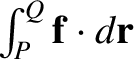.] Note that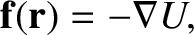(2.20)

irrespective of the path taken betweenand. Hence, it follows from Equation (2.18) that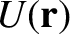(2.21)

for conservative forces. Another way of writing this is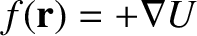(2.22)

Of course, we recognize Equation (2.22) as an energy conservation equation:is the object's total energy, which is conserved;is the energy the object has by virtue of its motion, otherwise known as its kinetic energy; and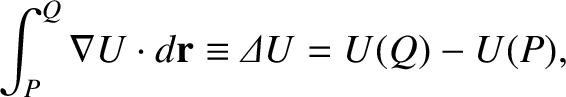is the energy the object has by virtue of its position, otherwise known as its potential energy. Note, however, that we can write energy conservation equations only for conservative forces. Gravity is an obvious example of such a force. Incidentally, potential energy is undefined to an arbitrary additive constant. In fact, it is only the difference in potential energy between different points in space that is well defined.Next: Newton's third law of Up: Newtonian mechanics Previous: Newton's first law of
Richard Fitzpatrick 2016-03-31